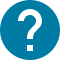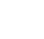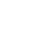大学院
HOME 大学院 Data Science for Practical Economic Research

UTASにアクセスできない方は、担当教員または部局教務へお問い合わせ下さい。

# Data Science for Practical Economic Research

Data Science for Practical Economic Research
Despite its name, this class is on forecasting methods in economics and applications of machine learning methods to forecasting. A typical class on machine learning focuses on cross-sectional data, leaving almost no space for a discussion of how to work with time series data and how to make forecasts with such data. The purpose of this class is to cover this gap. This class might be useful for students who plan to work at financial companies and government entities tasked with making forecasts. We will closely follow the textbook by G. Elliott and A. Timmermann "Economic Forecasting". The book is quite advanced and requires good understanding of probability and statistics. During the lectures, we will cover chapters from this textbook and perform hands-on sessions. All homework assignments for this class will be practical: students will be asked to apply methods covered in the class to real datasets. The required programming language is Python.

Students taking this class will be assumed to be familiar with basics of Machine Learning, probability and statistics, as well as programming in Python.

コース名

5123038
GPP-MP6E20L3
Data Science for Practical Economic Research
Kucheryavyy Konstantin
S1 S2

2

NO

Preliminary plan of lectures (subject to change): Lecture 1: Loss Functions. Lecture 2: The Parametric Forecasting Problem. Lecture 3: Classical Estimation of Forecasting Models; Bayesian Forecasting Methods. Lecture 4: Model Selection. Lecture 5: Univariate Linear Prediction Model. Lecture 6: Vector Autoregressions. Lecture 7: Forecasting in a Data-Rich Environment. Lecture 8: Nonparameteric Forecasting Methods. Lecture 9: Binary Forecasts. Lecture 10: Forecast Combinations. Lecture 11: Desirable Properties of Forecasts.

Lectures. Demo programs.

Grade will be based on homeworks.

– G. Elliott and A. Timmermann "Economic Forecasting" (Princeton University Press, 2006) - F.X. Diebold "Forecasting in Economics, Business, Finance and Beyond" (University of Pennsylvania, 2017) - Peter Fuleky (editor) "Macroeconomic Forecasting in the Era of Big Data: Theory and Practice" (Springer, 2020)

NA

- Good command of Python is assumed. - Students are assumed to be familiar with basics of Machine Learning.